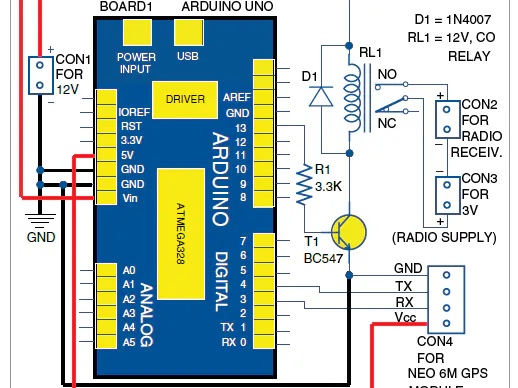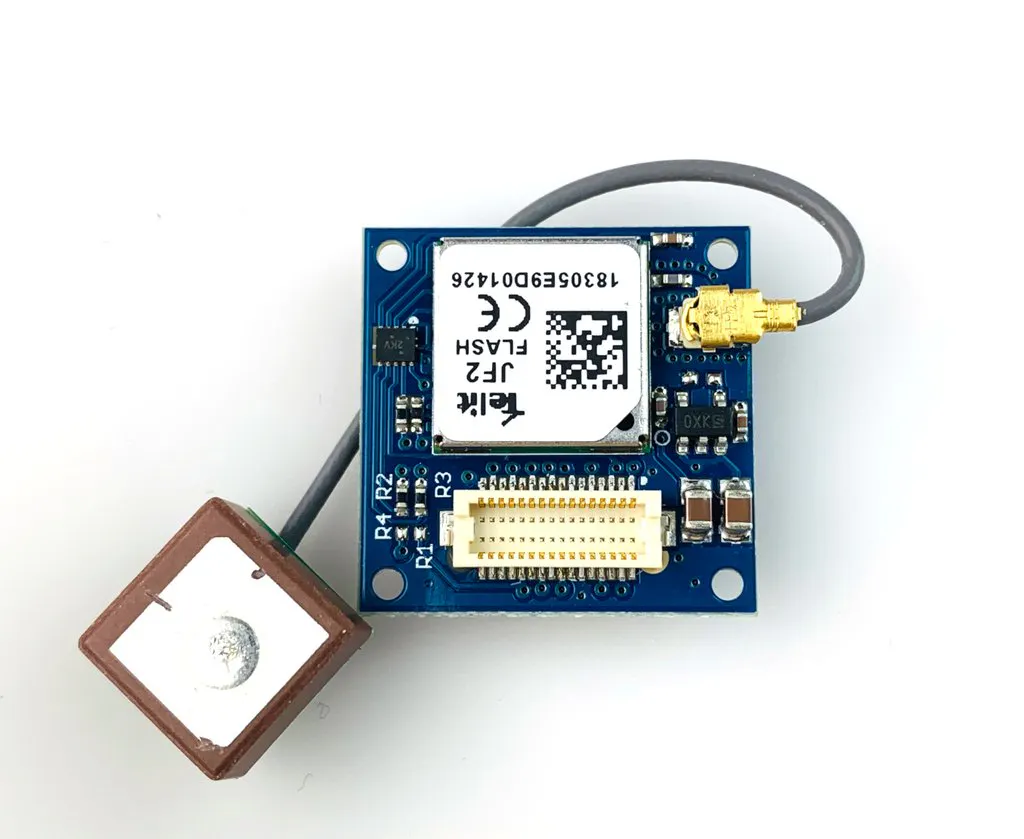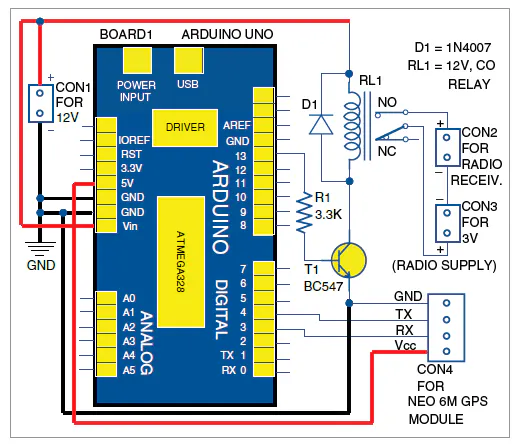# GPS based AIR News Broadcast System

GPS based Timer for broadcasting All India Radio ( Akashvani) at distress places where public are staying in LockDown.

IntermediateFull instructions provided1 hour695## Things used in this project

### Hardware componentsTinyCircuits TinyShield GPS
×1Arduino Nano R3
×1

## Schematics

### GPS based Timer IST Clock to turn on and off the Radio Set## Code

### GPS based Timer IST Clock

C/C++
Connect all parts as per circuit diagram and then upload the code.
```#include <TinyGPS++.h>
#include <SoftwareSerial.h>
/*
This sample sketch demonstrates the normal use of a TinyGPS++ (TinyGPSPlus) object.
It requires the use of SoftwareSerial, and assumes that you have a
4800-baud serial GPS device hooked up on pins 4(rx) and 3(tx).
*/
static const int RXPin = 4, TXPin = 3;
static const uint32_t GPSBaud = 9600;

// The TinyGPS++ object
TinyGPSPlus gps;

// The serial connection to the GPS device
SoftwareSerial ss(RXPin, TXPin);
//const int TimeZone = +5;
void setup()
{
Serial.begin(57600);
ss.begin(GPSBaud);
pinMode(13, OUTPUT);
}

void loop()
{
// This sketch displays information every time a new sentence is correctly encoded.
while (ss.available() > 0)
displayInfo();

if (millis() > 5000 && gps.charsProcessed() < 10)
{
Serial.println(F("No GPS detected: check wiring."));
while(true);
}
}
void displayInfo()// showing display to serial
{
delay(100);
//  Serial.print(F(" "));
if (gps.time.isValid())
{
int hr= (gps.time.hour()+5);
if(hr >12)
{
hr-=12;
}

int mn= (gps.time.minute()+30);
if(mn>59)
{
mn -=60;
}

int sec= (gps.time.second());
// display ////////////////////////////////////////////////
// if (hr < 9) Serial.print(F("0"));
if(gps.time.minute()>29 ) Serial.print (hr+=1);
else
{
Serial.print (hr);
}

Serial.print(F(":"));
if (mn < 10) Serial.print(F("0"));
Serial.print(mn);

Serial.print(F("\n"));
//////////////////////////////////// ADJUSTING TIMER AS PER SCHEDULE/////////////////
if ((hr == 6)or(hr == 7)or(hr == 8)or(hr == 9)or(hr == 10)or(hr == 11))
digitalWrite (13, HIGH);
if (mn > 9)
digitalWrite (13, LOW);
//////////////////////////////////////SPECIAL SCHEDULE////////////////////////////////////
if ((hr == 8) and (mn == 54))
digitalWrite (13, HIGH);
if ((hr == 8) and (mn == 55))
digitalWrite (13, HIGH);
// else
//  {
// digitalWrite (13, LOW);
//    }
}
}

```